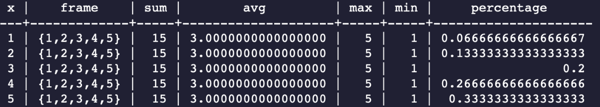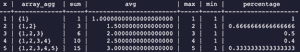Trusted answers to developer questions
Trusted Answers to Developer Questions

Related Tags

sql
postgresql

# What are aggregate window functions in SQL?

Ubaid Bakhtiar

Grokking Modern System Design Interview for Engineers & Managers

Ace your System Design Interview and take your career to the next level. Learn to handle the design of applications like Netflix, Quora, Facebook, Uber, and many more in a 45-min interview. Learn the RESHADED framework for architecting web-scale applications by determining requirements, constraints, and assumptions before diving into a step-by-step design process.

### Window functions in SQL

A window function in SQL is a very basic and essential utility. Window functions are a way to perform a variety of mathematical and analytical operations on a single row or multiple rows. The driving idea behind the purpose of the window function is to enable the user to work concurrently with multiple values of the result set. It is also the accessibility of window functions in SQL that makes it a very productive language to perform statistical operations like ranking and aggregates over a specific window (which we call frame).

Window functions work by accessing a specific window of the data in the input rows. We call this particular window frame.

SQL provides two major types of functions that can be performed on the selected frame. These functions are:

• Aggregate window functions

• Ranking window functions

We’ll discuss aggregate window functions in detail.

### Aggregate window functions

The functions that perform computations on multiple values/rows and return a single value are called aggregate window functions. Some of these functions are MIN(), MAX(), SUM(), COUNT() and AVERAGE().

We can use array_agg() as our aggregate function. It takes aggregates of the selected frame and outputs the content in the desired format. It has the following definition:

select x, array_agg(x) over (order by x)
Array_agg() definition

The over(order by x) actually means over (order by x rows between unbounded preceding and current row). We can also give the function definition as follows:

select x, array_agg(x) over (order by x rows between unbounded preceding and current row)
Another definition of array_agg()

We can also opt not to use a specific frame. In this case, the aggregate window function would have the following definition:

select x, array_agg(x) over() as frame
Opting a specific frame in array_agg() definiton

Let’s analyze an example that calculates the average and sum of the whole set of rows:

Performing Window Aggregate Functions with no frame specification*/select x,       array_agg(x) over () as frame,       sum(x) over () as sum,       avg(x) over () as avg,       max(x) over () as max,       min(x) over () as min,       x::float/sum(x) over() as percentage  from generate_series(1, 5) as t(x);
Example 1: Average and sum of the whole set of rows

The output of this sample would be as follows:Output of whole set of rows

We can see that the aggregate window function can very easily perform aggregate functions on a whole set of rows. We can easily do calculations like percentage and standard deviation using a whole set of rows.

We can also use frames to perform these functions on selected values in rows. The following example performs the same.

/* Performing Window Aggregate Functions with frame specification*/select x,       array_agg(x) over (order by x),       sum(x) over (order by x) as sum,       avg(x) over (order by x) as avg,       max(x) over (order by x) as max,       min(x) over (order by x) as min,       x::float/sum(x) over(order by x) as percentage  from generate_series(1, 3) as t(x);
Example 2: Average and sum of a selected set of rows

The output would be as follows:Output on selected set of rows

### Try it yourself

We can also try to implement different window aggregate functions in the playground provided below:

select x,       array_agg(x) over () as frame,       sum(x) over () as sum,       avg(x) over () as avg,       max(x) over () as max,       min(x) over () as min,       x::float/sum(x) over() as percentage  from generate_series(1, 5) as t(x);
No frame aggregate window function

In this exercise, we see that the sum, average, maximum, minimum, and percentage operations are performed on the whole set of rows. This method is useful if we want to calculate the sum, average, maximum, and minimum.

select x,       array_agg(x) over (order by x),       sum(x) over (order by x) as sum,       avg(x) over (order by x) as avg,       max(x) over (order by x) as max,       min(x) over (order by x) as min,       x::float/sum(x) over(order by x) as percentage  from generate_series(1, 5) as t(x);
Specific frame aggregate window function

This method is also useful if we want to calculate the percentage fractions.

RELATED TAGS

sql
postgresql

CONTRIBUTOR

Ubaid Bakhtiar